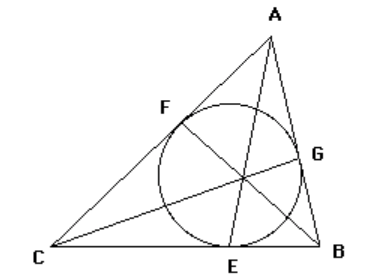# Gergonne's TheoremThe incircle of triangle $ABC$ touches the side $BC, AC$ and $AB$ at $D, E$ and $F$.

Then the lines $AE, BF$ and $CG$ are concurrent.

Their meeting point is called the Gergonne point.